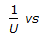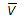# Chemical Engineering - Heat Transfer

### Exercise :: Heat Transfer - Section 8

1.

The type of liquor circulation system to be ' employed in evaporators (viz. natural or forced circulation) is determined mainly by the __________ of the liquid.

 A. viscosity B. density C. thermal conductivity D. corrosive nature

Answer: Option A

Explanation:

No answer description available for this question. Let us discuss.

2.

In case of surface condensers, a straight line is obtained on plotting__________ on an ordinary graph paper.

 A. 1/-0.8 B.-0.8 C.-2 D. 1/-2

Answer: Option A

Explanation:

No answer description available for this question. Let us discuss.

3.

Thermal diffusivity is the most important in heat transfer by

 A. conduction B. radiation C. condensation D. natural convection

Answer: Option A

Explanation:

No answer description available for this question. Let us discuss.

4.

(NGr x NPr) is called the __________ number.

 A. Graetz B. Reyleigh C. Nusselt D. Stanton

Answer: Option B

Explanation:

No answer description available for this question. Let us discuss.

5.

A dephlegmator is a

 A. total condenser B. vacuum evaporator C. partial condenser D. double pipe heat exchanger

Answer: Option C

Explanation:

No answer description available for this question. Let us discuss.

#### Current Affairs 2021

Interview Questions and Answers